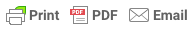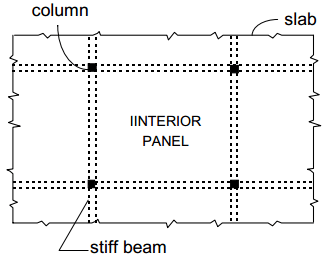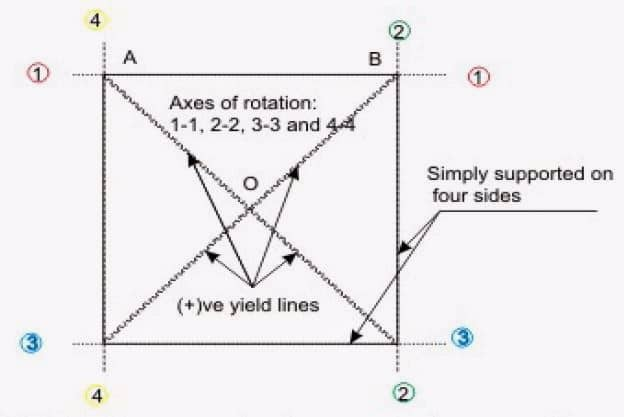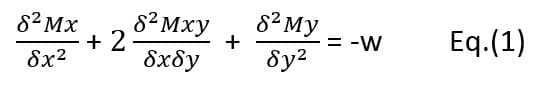Sorry, you do not have permission to ask a question, You must login to ask question. Become VIP Member

Do you need to remove the ads? Become VIP Member

## What is Yield Line Theory?

The yield line theory of analysis  is a factored or ultimate load method of analysis. The yield line theory is conducted based on the bending moment of the structural element at its collapse state.

The yield analysis was proposed by Ingerslev in 1923. The importance of the theory is more reflected in the analysis of slabs.

## Yield Lines in Concrete Slabs

As mentioned before, the analysis is conducted based on the collapse load of the slab under consideration. Under this load, cracks are formed. This crack formation is seen in under reinforced concrete slabs and the yield line theory considers only under reinforced concrete slabs.

During the crack formation in the slabs, the reinforcement starts to yield. This yielding happens at the point of maximum bending moments. As the cracks propagates, the yield lines started to develop. Finally, under uncontrollable load, the slab will collapse leaving maximum yield line representing the achievement of maximum bending moments. The main principle of yield line theory is to determine the location of appropriate yield lines.

In the case of two-way slab systems, which are statically indeterminate, detailed inelastic analysis (yield line analysis) is conducted. The moment coefficients given in the Code IS 456:200(Table 26) for two-way rectangular slabs with various possible edge conditions are based on Yield Line Analysis. ‘Yield line analysis’ is the equivalent for a two-dimensional flexural member (plate or slab) of the limit analysis of a one-dimensional member (continuous beam).

## Characteristics of Yield Lines in R.C Slabs

The following are the characteristics of yield lines formed in reinforced concrete slabs under ultimate loads:

i) Yield lines are straight

ii) Yield lines end at supporting edges of slab& Ad Free!

GET VIP MEMBERSHIP NOW!

iii) Yield lines passes through intersection of axis of rotation of adjacent slab elements

iv) Axis of rotation lies along lines of supports and passes over columnsFig.1: Typical interior panel in a two-way slab systemFig.2: Yield line pattern under uniformly distributed collapse loadFig.3: Deflected shape at collapse (section A-A)

## Assumptions of Yield Line Theory

The following are the assumptions of the yield line analysis of reinforced concrete slabs:

1. At the collapse stage, the steel reinforcement will be fully yielded along the yield lines.
2. During the collapse, the slab is deformed plastically and they gets separated into segments. Elastic behaviour is followed by the individual segments.
3. In yield theory, only plastic deformations are taken into consideration. The elastic deformations are neglected. The so called deformations are happening along the yield lines. Even in the collapse state, the elements will remain plane.
4. Along the yield lines, there is uniform distribution of bending and twisting moments. The amount of reinforcement in the section will reflect the capacity that will give the maximum moment capacity of the section.
5. The yield lines are lines of intersection of two planes, hence they will remain straight.

## Rules of yield lines

The  positive and negative yield lines, are used to designate the yield lines for positive bending moments having tension at the bottom and negative bending moments having tension at the top of the slab, respectively.

The guidelines for predicting the yield lines and axes of rotation are mentioned below:

1. Straight yield line occure between two intersecting planes.
2. In one way slabs, the positive yield line occur at the mide span.
3. At the supports, the yield lines are negative in addition to the mid positive yield lines for one way continuous slabs.
4. For slabs with a point load, the yield lines will be projecting out of the point of application.
5. For two planes intersecting, the yield line will pass through the intersection of axes of rotation of two planes.
6. The end of yield lines are either at the boundary of the slab or at another yield line.
7. The axes of rotation is represented by yield lines
8. The edges of support too act as axes of rotation. If the support is fixed, the support will have negative yield lines. This negative yield line will have resistance to rotation. In the case of simply supported edges, the axes of rotation at the support will not have resistance to rotation.
9. For a column support, the axis of rotation pass over it. The orientation will depend on other considerations

## Methods of Analysis in Yield Line Theory

Based on the rules and the assumptions, a general load pattern and the axis of rotation is located. The below mentioned methods can be used to determine the final pattern, axes of rotation and the collapse load. The methods used are:

1. Method of Segmental Equilibrium
2. Method of Virtual Work

### 1.Methods of Segmental Equilibrium

As the name says, the collapse of the slab under certain mechanism will give several segments. The equilibrium of the segments is taken into consideration and hence to arrive at set of simultaneous equations.

The solution of the simultaneous equations will give certain parameter values that will help us to finalise the yield pattern. This will also give us a relation between the load capacity and the moment value.

As shown in figure-4, each segment is studied as a free body. Each segment is under equilibrium under the action of moment, loads along the yield lines. The reactions and shear will be along the supports. Here, the twisting moments along the yield lines will be zero.

From figure-4,Considering segments AOB, about axis of rotation 1-1Fig.4:Yield Line Pattern of a Two Way Simply Supported Slab

The vector sum of moments along AO & OB = Moments due to the loads on the segment AOB

For a small element of length dx and dy, the governing equation of equilibrium is given:Here,

External load acting per unit area = w

Bending Moments per unit width x and y = Mx & My

Twisting Moment = Mxy

### 2.Method of Virtual Work

The principle of virtual work is followed in this method. Virtual Work principle states that,

The external work done by the loads to cause small virtual deflection is equal to the small internal work done by the yield moments to make a rotation to accommodate the virtual deflection.

Here, the slab is assumed with a yield pattern and axes of rotation. The system is in equilibrium with the moments and loads acting on it. This system is subjected to an infineitesimal increase in load so that the structure will undergo further delflection.

Based on the principle, the internal and the external works is equated hence giving a relationship between the applied loads and the ultimate resisting moments. The work equation is given by:

###Where,

Vertical Deflection along which load “w” moves = D

Moment capacity per unit length of the section = M

Rotation of the slab segment = ?

Length of Yield Line = l

EDITOR